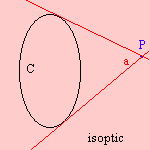# isoptic

## mainLet's have a curve C. Vary two tangents to the curve in such a way that the angle between the two lines is a constant. Then the intersections of the tangents form the isoptic of C.
The sinusoidal spiral is the curve for which the isoptic is also a sinusoidal spiral.

Some isoptic curves are the following:

 curve isoptic cycloid trochoid epicycloid epitrochoid hypocycloid hypotrochoid parabola hyperbola

When the constant angle between the tangents has the value π/2, the curve is called an orthoptic.

For the logarithmic spiral its orthoptic is equal to itself.

Some other orthoptic curves are:

 curve orthoptic astroid quadrifolium cardioid limaçon deltoid circle ellipse hyperbola parabola directrix

La Hire (1704) was the first to mention the curve.

Another curve with isoptic properties is the isoptic cubic.